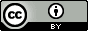## Thursday, December 27, 2012

### Marcel Swart - I am a (computational) chemist

Very nice 20 min presentation to non-specialists by computational chemist Marcel Swart.

## Wednesday, December 19, 2012

### Molecular Modeling Basics Reviewed in Journal of Chemical Education

A very nice review of the book (and mention of this blog) by John Shibata in J. Chem. Ed.  Much appreciated!

This website allows you to draw a ligand and dock it to one of 10,000 target proteins.  You can read a bit more about it here on their blog.

## Sunday, December 16, 2012

### Conformational and rotational entropy and point group symmetry

The point group affects the free energy
The use of point group symmetry in quantum chemical calculations can speed up calculations significantly, but it is often difficult to input symmetric coordinates correctly so many people opt to run calculations without symmetry (i.e. in $C_1$ symmetry) anyway.  However, the lack of symmetry changes the rotational entropy and, hence, the free energy you compute. So if you have symmetric molecules but choose to run in $C_1$ symmetry you must correct the entropies and free energies.

The rotational entropy is given by$$S_{rot}=R\ln\left(\frac{8\pi^2}{\sigma}\left(\frac{2\pi ekT}{h^2}\right)^{3/2}\sqrt{I_1I_2I_3}\right)$$ $\sigma$ is called the symmetry number and depends on the point group: for example, $\sigma=1$ for $C_1$ and $C_s$, $\sigma=n$ for $C_{nv}$ and $C_{nh}$, $\sigma= 2n$ for $D_{nh}$ and $D_{nd}$, and $\sigma= 12$ or $T_d$.  So the rotational entropy calculated with and without symmetry will differ by $R\ln(\sigma)$:$$S_{rot}=S_{rot}^{C_1}-R\ln(\sigma)$$and similarly for the free energy$$G^\circ=G^{\circ,C_1}+RT\ln(\sigma)$$
An example: $H_2O+Cl^-\rightleftharpoons HOH\cdots Cl^-$
The free energy change for this reaction is$$\Delta G^\circ=\Delta G^{\circ,C_1}+RT\ln\left(\frac{\sigma_{HOH\cdots Cl^-(C_s)}}{\sigma_{H_2O(C_{2v})}\sigma_{Cl^-(C_1)}}\right)\\ \Delta G^\circ=\Delta G^{\circ,C_1}+RT\ln\left(\frac{1}{2\times 1}\right)=\Delta G^{\circ,C_1}-RT\ln (2)$$The corresponding equilibrium constants are$$K=e^{-\Delta G^\circ/RT}=2K^{C_1}$$So, $\sigma$'s accounts for the fact that, because of the symmetry of water, there are two ways of making $HOH\cdots Cl^-$and another way to view $R\ln(2)$ is that it is the conformational entropy of the complex.

A test: $H_2O+NH_3\rightleftharpoons HOH\cdots NH_3$
For the above equilibrium what is $X$ in$$\Delta G^\circ=\Delta G^{\circ,C_1}-RT\ln (X)$$

An exception: $2H_2O\rightleftharpoons HOH\cdots OH_2$
Based on the rules outlined so far one would expect the free energy change for this equilibrium to be$$\Delta G^\circ=\Delta G^{\circ,C_1}-RT\ln (4)$$The factor of four accounts for the fact that there are four ways of making $HO^AH\cdots O^BH_2$. However, since the water molecules are identical there are actually four additional water dimer possibilities for  $HO^BH\cdots O^AH_2$, so$$\Delta G^\circ=\Delta G^{\circ,C_1}-RT\ln (8)$$In general, for $A+A\rightleftharpoons Product$ reactions the symmetry number for $A+A$ is $2\sigma_A^2$rather than $\sigma_A^2$

All these considerations also apply to activation free energies and rate constants as outlined in this excellent paper by Fernández-Ramos et al., which inspired this post.  See also this excellent paper by Gilson and Irikura.## Wednesday, December 12, 2012

### Thermodynamics in solution: a brief guide for quantum chemists

2015.01.25: Please read this paper instead of this post. It turns out most of what I write here is wrong.

The thermodynamic properties such as enthalpy, entropy, and free energy you get from a vibrational analysis by programs such as GAMESS and Gaussian are those of an ideal gas at 1 bar pressure (and usually 298 K).  If you calculate free energy changes in solution (using methods such as PCM or COSMO) there are a few things you should do differently compared to gas phase calculations.  These things will only make a difference if you are computing free energy changes for processes where the number of particles change, such as binding free energies.  The corrections will cancel out for things such as conformational free energy differences.

Use Helmholtz free energies instead of Gibbs free energies
Experimental studies typically report Gibbs free energy changes ($\Delta G^\circ$), which are related to Helmholtz free energy changes ($\Delta A^\circ$) by$$\Delta G^\circ = \Delta A^\circ + p^\circ \Delta V$$ $\Delta V$ is the change in volume of the solution due to the reaction.  This volume change is negligible so $\Delta G^\circ = \Delta A^\circ$ is a good approximation.

The Gibbs free energy printed by quantum chemistry program correspond to an ideal gas where $pV=RT$ and the difference between $\Delta G^\circ$ and $\Delta A^\circ$ is much larger.

Computing free energies with semi-empirical methods
Most semiempirical methods such as AM1 and PM6 are parameterized such that the electronic energy matches experimental heat of formations ($\Delta H_f^\circ$) at 298 K.  So a gas phase free energy change should be computed as$$\Delta G^\circ=\Delta\Delta H_f^\circ-T\Delta S^\circ$$i.e. you don't need any of the enthalpy information printed out as part of the vibrational analysis.  A solution free energy change should be computed as $$\Delta A^\circ=\Delta\Delta U_f-T\Delta S^\circ$$where $\Delta U_f=\Delta H_f^\circ-RT$

Added 2013.08.16: However, dispersion and/or hydrogen bond corrected semi-empirical methods such as PM6-DH+ are parameterized against electronic binding energies.  So if you are computing binding free energies with such methods you need to add the translational, rotational, and vibrational enthalpy corrections to  $\Delta\Delta H_f^\circ$.

Change the standard state to 1 mol per liter
The translational entropy printed out depends on the volume of the system, which is computed as $V=RT/p$ = 24.79 liters.  For solution that volume should be 1 liter.  Most programs do not allow you to change volume so you must apply the correction to the entropy$$S_{soln}^\circ=S_{gas}^\circ+R\ln\left(\frac{1}{24.79}\right)$$ yourself

Is it OK to use the rigid rotor-harmonic oscillator approximation in solution?
Short answer: yes.  The derivations of the translational, rotational, and vibrational free energies are done for an isolated molecule in vacuum, while a molecule in solution interacts with solvent molecules.  However, a molecule in solution still has the exact same degrees of freedom as in the gas phase: it is free to explore the entire three dimensional volume and is free to adapt any rotational angle just like in the gas phase, so the resulting free energy expressions are the same.  Similarly, the individual molecules still have $3N-6$ internal vibrational frequencies.  The energy from the solute-solvent vibrations are included in the solvation free energy.

This blogpost was inspired by this excellent paper by Muddana and Gilson, and I thank Mike Gilson for helpful discussions.